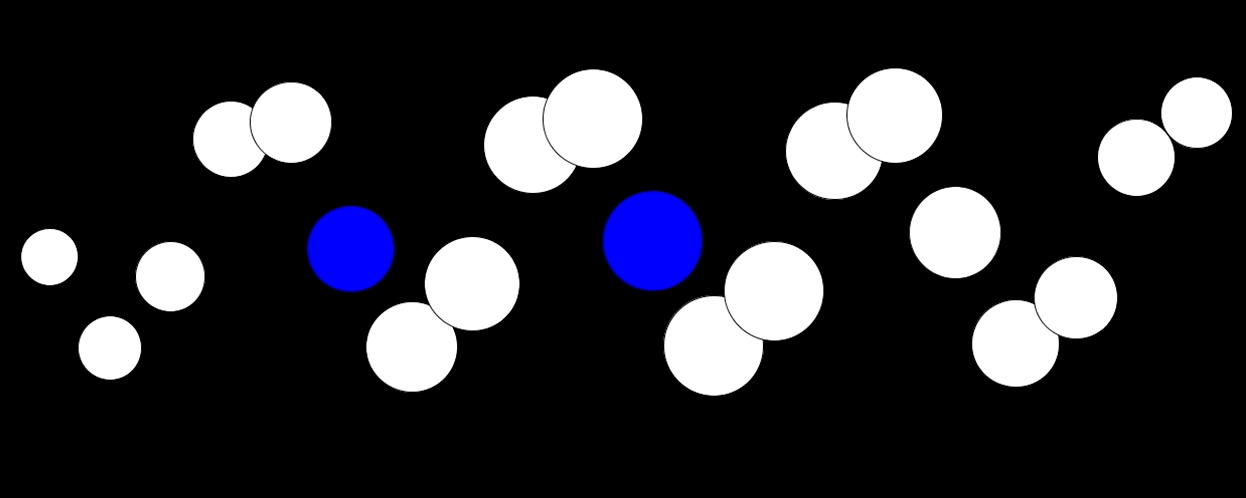# Processing

I’ve recently been playing with Processing. Here are the results. I did experiment with triggering some MIDI notes at the center threshold but it didn’t sound as good as I wanted. I’ll expand on that once I get a bit more time.

## The Source

```import themidibus.*; //Import the library
MidiBus myBus; // The MidiBus
float b = 20;
float d = 0;

void setup () {
size(1280, 720);
frameRate(30);
MidiBus.list();
myBus = new MidiBus(this, "Bus 1", "Bus 1");
}

void draw() {

int icCount = 20;
double number = Math.PI * 2 / icCount;
background(0);

for (int n=1; n<= icCount; n++) { double x = 300 + ((Math.sin(number * (17-(n/ Math.PI)) * b)) * 120); double y = (n * 60) + 20; double s = 50 * (Math.sin(b*n) +1); //if(n % 4 ==0) { double check = Math.sin(number * (17-(n/ Math.PI)) * b); if (check >=0.1 && check <=0.2) {
//myBus.sendNoteOn(1, n + 60, 12);
fill(#0000ff);
} else {
//myBus.sendNoteOff(1, n + 60, 12);
fill(#ffffff);
}
//}

ellipse((float)y,(float)x,(float)s,(float)s);
}

b += 0.02;
d += 0.04;

}
```

Check out the software here: https://processing.org/
Check out other sine programming examples here (JavaScript): https://roguelj.co.uk/toybox/